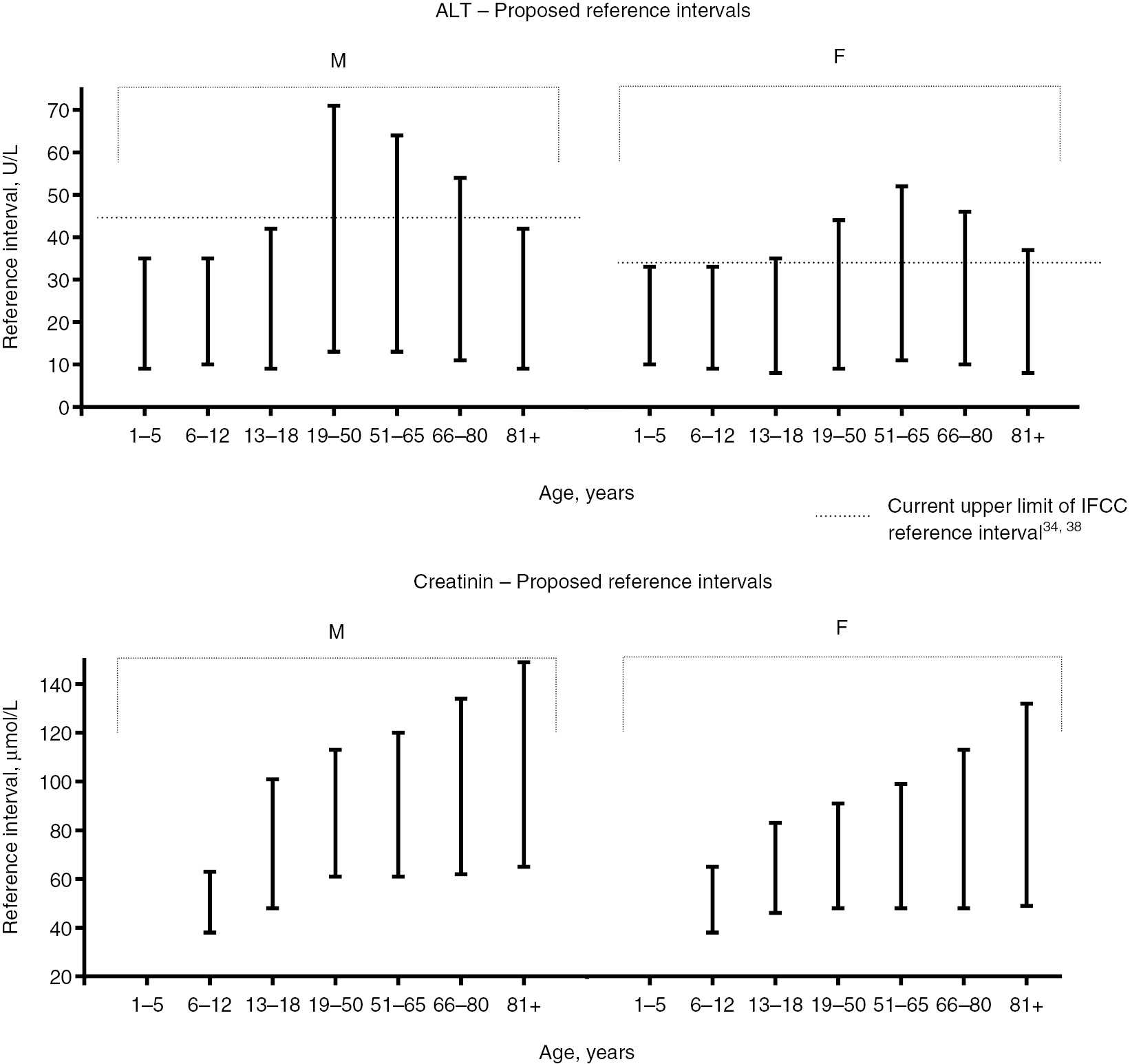## Basic concepts for reference intervals

#### Example of reference intervals for creatinine as a function of age and sex## Comparison of methods for obtaining a reference interval

As presented in the Concepts section, there are different methods for obtaining a reference interval. In this section, you can explore the results on samples of a normal distribution defined by its mean and sd. and compare the theoretical reference interval with the estimated intervals in the different methods.

### Population parameters

A sample is randomly simulated from a normal distribution with the selected parameters. The reference interval are computed by three methods.

### Compute the reference interval from a sample of a normal distribution

Here we consider you can indicate the mean and standard deviation of a sample for a biomarker of interest. Then, you can compute the corresponding reference interval using the Student's method. The results also show the 95% confidence interval of the reference interval limits. In the figure, we show thereference interval by a red line and the confidence interval for each limit in blue. In this case, we assume the sample comes from a normal distribution.

## Read an EXCEL file and compute the reference interval of one variable

You can select an EXCEL file by navigating thorough your file system. Then, you can visualize the variables in that file, select one and obtain the reference interval. As a disgnostic tool, we include the histogram of the selected variable an a QQ-plot for evaluating if the normal distribution is an appropriate model for that variable.

If the sample shows a clear non-normal aspect in its distribution, the Student's method results are not valid. In that case the CI of the ref.int. limits are also non valid.

##### First cases of the selected variable...